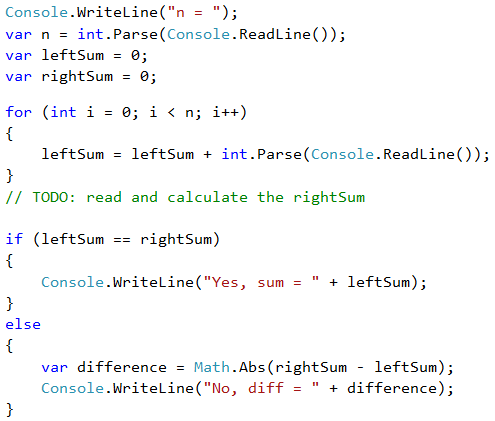# Problem: Left and Right Sum

Write a program that converts 2 * n integers and checks whether the sum of the first n integers (left sum) equals the sum of the second n numbers (right sum). In case the sums are equal, print "Yes" + the sum, otherwise print "No" + the difference. The difference is calculated as a positive number (by absolute value). The format of the output must be identical to the one in the examples below.

## Sample Input and Output

Input Output Input Output
2
10
90
60
40
Yes, sum = 100 2
90
9
50
50
No, diff = 1

## Video: Left and Right Sum

Watch this video lesson to learn how to calculate the left and the right sum and their difference: https://youtu.be/s_uAuqTnC8w.

## Hints and Guidelines

We will first input the number n, after that the first n numbers (left half) and sum them up. We will then proceed with inputting more n numbers (the right half) and sum them up. We calculate the difference between the sums by absolute value: Math.Abs(leftSum - rightSum). If the difference is 0, print "Yes" + the sum, otherwise print "No" + the difference.